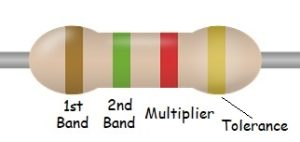Identifying resistor values can be tricky. We created this easy resistor value calculator to help you quickly determine the values of your standard resistors. Calculating the value is pretty simple, memorizing the color code values is not for most of us!

• For 3 & 4 band resistors, the first 2 bands are always the value digits. While for 5 & 6 bands the first 3 are the value. The values form a whole number, a 4 band resistor with RED RED would be 22
• The next band after value digits is always the multiplier. This value multiplied by the first 2 or 3 bands and is your resistance value in Ohms.
• With \$ & 5 band resistors the last band tells you the Tolerance of your resistor, +/- x% meaning the actual resistance may vary plus or minus x%, the tighter the tolerance the more accurate your component will be (and probably a bit more expensive per piece.)
• If a 6th Band is present it indicates the Temperature Coefficient. How your resistance value may change +/- over temperature ranges. Understanding this is a story for another day…
Let’s look at an example:This is a 4 Band resistor, so to get the base value we take the first 2 bands, BROWN-1, GREEN-5, giving us 15. The multiplier band is RED-2 which is 100 Ohms. To get the actual value of the resistor we take 15 x 100 ohms = 1500 Ohms or 1.5K Ohms. The last band, GOLD indicates the Tolerance +/- 5%. It’s not very complicated once you’ve memorized the color values. Try it down below!

So if you are like me and have a hard time remembering all the colors and their values, use this simple calculator below to find out the values of your resistor.

Resistor Calculator

Select the total number of bands on your resistor